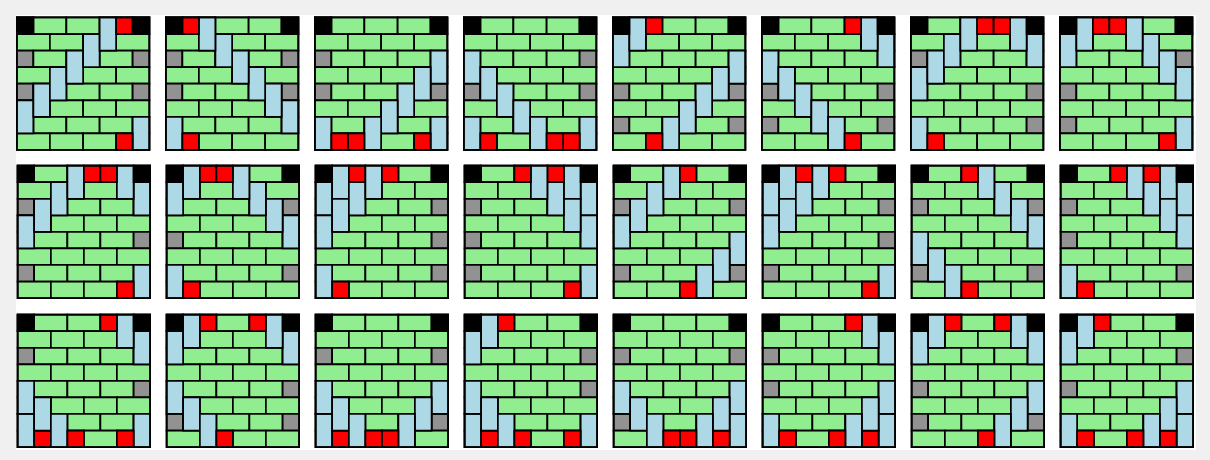## Enumerating tatami mat arrangements of square grids with $v$ vertical dimers

Alejandro Erickson, Department of Computer Science, University of Victoria, Canada.
Frank Ruskey, Department of Computer Science, University of Victoria, Canada.

### Abstract:

Let $\mathbf{T}_{n}$ be the set of monomer-dimer tatami tilings of the $n\times n$ grid with the maximum number, $n$, of monomers, and which have a monomer in each of the top left and top right corners. We consider those tilings of $\mathbf{T}_n$ with exactly $v$ vertical dimers and prove that their numbers are the coefficients of the polynomial \begin{align} \label{eq:tnzIntro} V\hspace{-5pt}H_n(z):=2\sum_{i=1}^{\lfloor (n-1)/2 \rfloor} S_{n-i-2}(z)S_{i-1}(z)z^{n-i-1} + \left(S_{\lfloor (n-2)/2 \rfloor}(z)\right)^2, \end{align} where $S_n(z)$ is the generating function of the number of subets of $\{1,2,\ldots, n\}$ whose members sum to $k$. Furthermore, $V\hspace{-5pt}H_h(z)$ has the factorization \begin{align*} P_n(z)\prod_{j\ge 1} S_{\lfloor (n-2)/2^j \rfloor}(z), \end{align*} where $P_n(z)$ is a somewhat mysterious polynomial with several observable patterns. We show for all $n\ge 2$, that $P_n(1) = n2^{\nu(n-2)-1}$, where $\nu(n)$ is the number of $1$s in the binary representation of $n$ and that $\deg(P_n(z)) = \sum_{k=1}^{n-2} \text{Od}(k)$, where $\text{Od}(k)$ is the largest odd divisor of $k$. In addition $P_n(z)$ is irreducible and \begin{align*} \sum_{n\ge 2} P_n(-1)z^{n-2} = \frac{(1+z)(1-2z)}{(1-2z^2)\sqrt{1-4z^2}}, \end{align*} at least for $2\le n < 200$. The combinatorial decomposition underlying the polynomial $V\hspace{-5pt}H_n(z)$ leads to an algorithm for exhaustively generating these tilings in constant amortized time per tiling.

Below we show all tilings in $\mathbf{T}_8$ with $7$ vertical dimers.The plots below show the roots of the polynomial $P_n(z)$ for $n$ even on the left and for $n$ odd on the right. In both cases $3 \le n \le 49$. Darker and smaller points are used for larger values of $n$. Clicking on the image will bring up a (much) larger image of the respective plot.